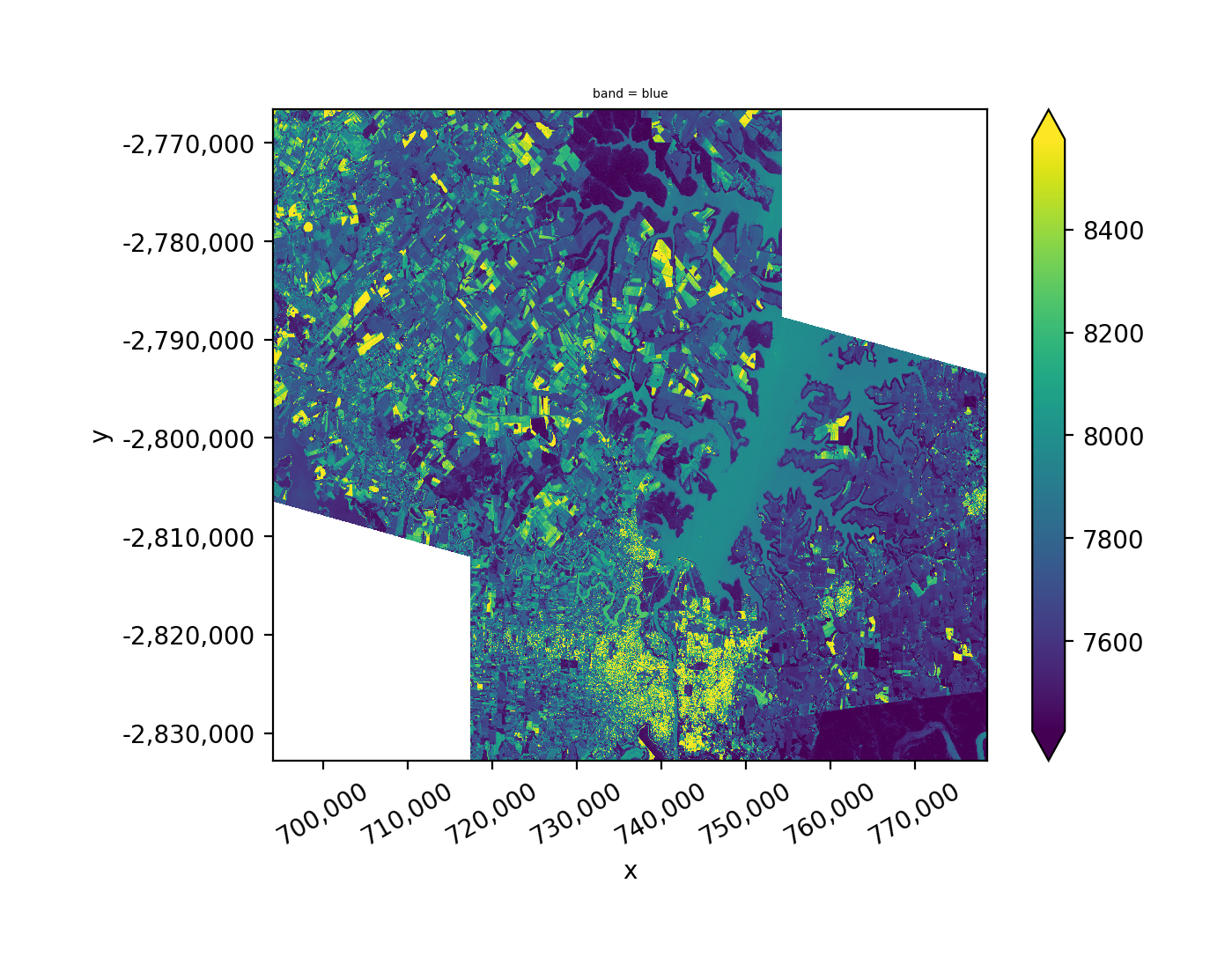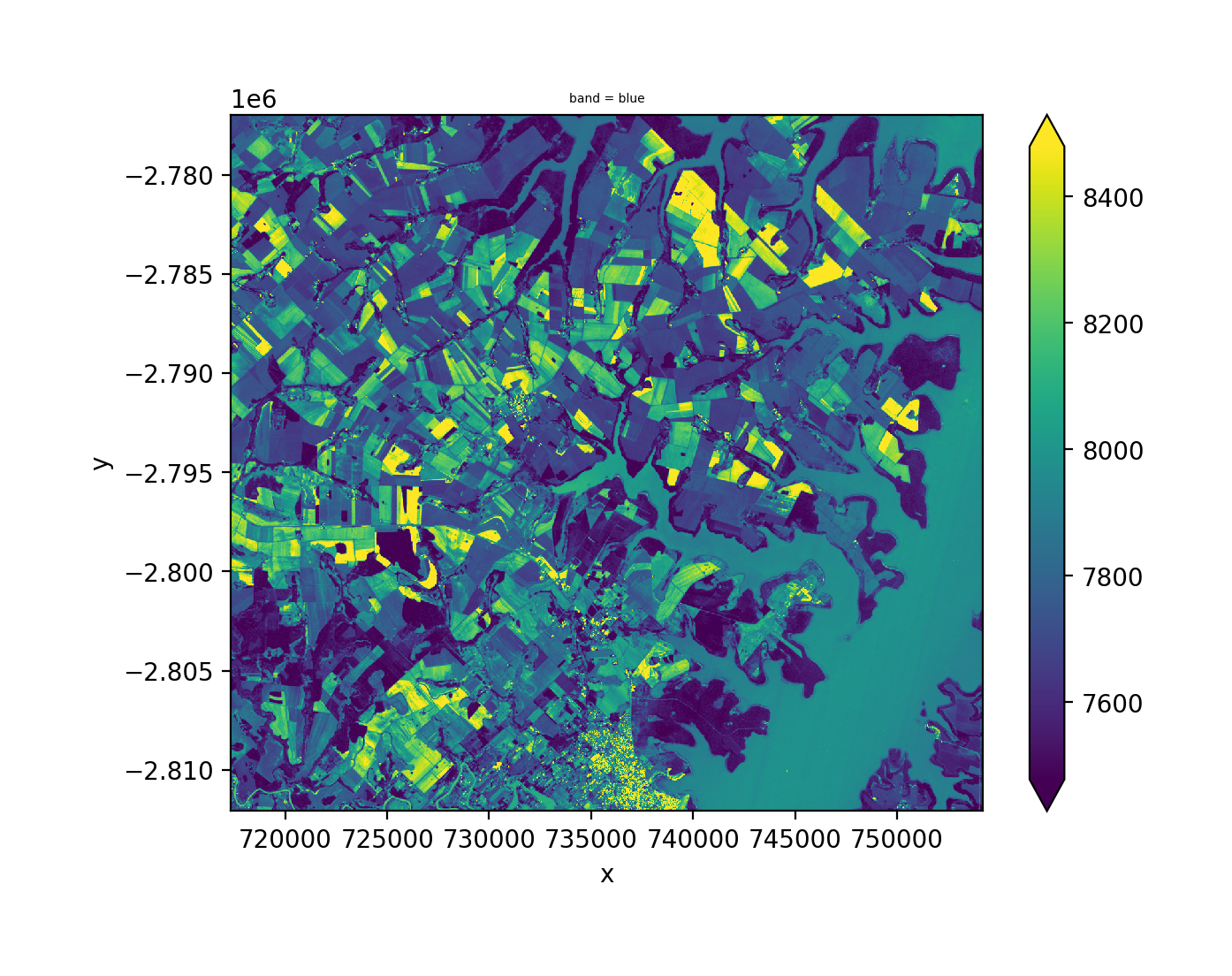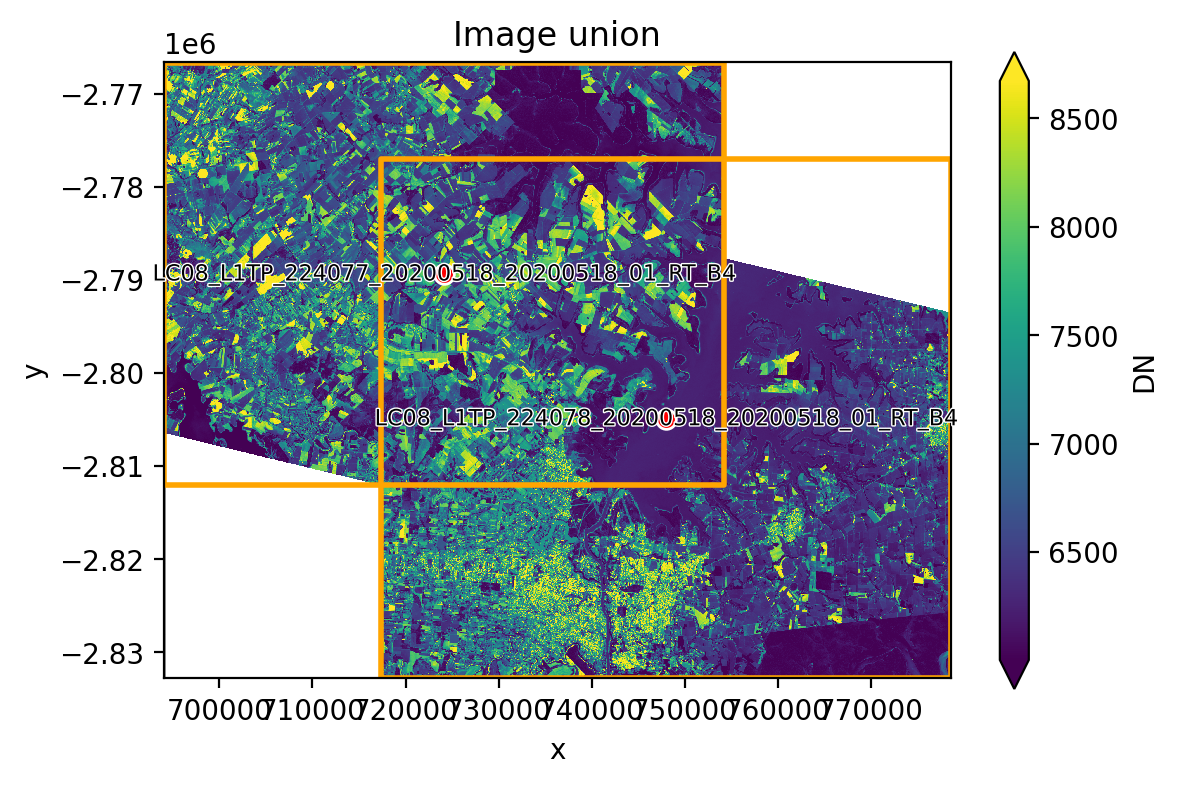Learning Objectives

• Create mosaics of more than one multiband image

• Find the intersection of two images

• View the footprint of multiple image tiles

Review

# Handle Multiple Remotely Sensed Images#

Doing analysis over larger areas often requires the use of image mosaics (combining two or more images). Luckily for us geowombat makes this process relatively easy.

## Union (Mosaic) of Remotely Sensed Image#

As an example let’s plot the union with mosaic=True of two images taken on the same day, for the overlapping portions we will use the mean pixel value by setting overlap='mean', but blue band only. Alternatively we could use one of ‘mean’, ‘min’, or ‘max’.

Note we rename the band name with band_names=['blue'].

# Import GeoWombat
import geowombat as gw

# import plotting
import matplotlib.pyplot as plt
import matplotlib.patheffects as pe

from geowombat.data import l8_224077_20200518_B2, l8_224078_20200518_B2

fig, ax = plt.subplots(dpi=200)
filenames = [l8_224077_20200518_B2, l8_224078_20200518_B2]
with gw.open(filenames,
band_names=['blue'],
mosaic=True,
overlap = 'mean',
bounds_by='union') as src:
src.where(src != 0).sel(band='blue').plot.imshow(robust=True, ax=ax)## Intersection of Remotely Sensed Image#

Same idea with the intersection, using bounds_by='intersection', we still need to mosaic the two images mosaic=True.

fig, ax = plt.subplots(dpi=200)
filenames = [l8_224077_20200518_B2, l8_224078_20200518_B2]
with gw.open(filenames,
band_names=['blue'],
mosaic=True,
overlap='mean',
bounds_by='intersection') as src:
src.where(src != 0).sel(band='blue').plot.imshow(robust=True, ax=ax)## View Image Tile Footprints#

Here we set up a more complicated plotting function for near IR ‘nir’. Note the use of footprint_grid.

from geowombat.data import l8_224077_20200518_B4, l8_224078_20200518_B4

def plot(bounds_by, ref_image=None, cmap='viridis'):
fig, ax = plt.subplots(dpi=200)
with gw.config.update(ref_image=ref_image):
with gw.open([l8_224077_20200518_B4, l8_224078_20200518_B4],
band_names=['nir'],
chunks=256,
mosaic=True,
bounds_by=bounds_by) as srca:
# Plot the NIR band
srca.where(srca != 0).sel(band='nir').plot.imshow(robust=True, cbar_kwargs={'label': 'DN'}, ax=ax)
# Plot the image chunks
srca.gw.chunk_grid.plot(color='none', edgecolor='k', ls='-', lw=0.5, ax=ax)
# Plot the image footprints
srca.gw.footprint_grid.plot(color='none', edgecolor='orange', lw=2, ax=ax)
# Label the image footprints
for row in srca.gw.footprint_grid.itertuples(index=False):
ax.scatter(row.geometry.centroid.x, row.geometry.centroid.y,
s=50, color='red', edgecolor='white', lw=1)
ax.annotate(row.footprint.replace('.TIF', ''),
(row.geometry.centroid.x, row.geometry.centroid.y),
color='black',
size=8,
ha='center',
va='center',
path_effects=[pe.withStroke(linewidth=1, foreground='white')])
# Set the display bounds
ax.set_ylim(srca.gw.footprint_grid.total_bounds-10, srca.gw.footprint_grid.total_bounds+10)
ax.set_xlim(srca.gw.footprint_grid.total_bounds-10, srca.gw.footprint_grid.total_bounds+10)
title = f'Image {bounds_by}' if bounds_by else str(Path(ref_image).name.split('.')) + ' as reference'
size = 12 if bounds_by else 8
ax.set_title(title, size=size)

The two plots below illustrate how two images can be mosaicked. The orange grids highlight the image footprints while the black grids illustrate the DataArray chunks.
plot('union')